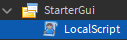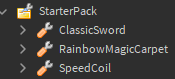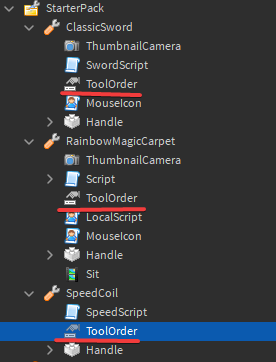# How To Make A Scrollable Inventory System

## How To Make A Scrollable Inventory

Hi there! In this post, I’ll be showing you how to make a scrollable inventory (with CoreGui inventory disabled). Kind of like the inventory system made on Arsenal, and other FPS shooter games.

I haven’t really seen any tutorials on DevForum about this, so I decided to make this post. If you have any criticism or recommendations for the code, feel free to tell me! Anyways, without further ado, let’s start.

## Step 1:

Create a local script in StarterGui, this is where we’ll do all of the code for the items.## Step 2:

Add all of your tools that you want included in the inventory system inside StarterPack.## Step 3:

Add a NumberValue inside each tool, name it “ToolOrder” and set each value to 1, then 2, then 3. (Example: ClassicSword value = 1, RainbowMagicCarpet value = 2, SpeedCoil value = 3)

This is so that we can sort through the tools inside the local script.## Step 4:

Insert this code inside the local script:

SortTools() checks for the number value “ToolOrder”, and equips a tool based on the direction of the mouse wheel.

``````local UIS = game:GetService("UserInputService")
local PS = game:GetService("Players")

local playerObject = PS.LocalPlayer
local character = playerObject.Character or playerObject.CharacterAdded:Wait()
local humanoid = character:WaitForChild("Humanoid")
local currentOrder = 0

local debounce = false

local function SortTools(key, tools) -- SORTS THE TOOLS
local toolOrder = tools:FindFirstChild("ToolOrder")

if key == "up" then
if currentOrder == 0 and toolOrder.Value == 1 then
humanoid:EquipTool(tools)
currentOrder = toolOrder.Value

elseif currentOrder == 1 and toolOrder.Value == 2 then
humanoid:EquipTool(tools)
currentOrder = toolOrder.Value

elseif currentOrder == 2 and toolOrder.Value == 3 then
humanoid:EquipTool(tools)
currentOrder = toolOrder.Value

elseif currentOrder == 3 and toolOrder.Value == 1 then
humanoid:EquipTool(tools)
currentOrder = toolOrder.Value
end
elseif key == "down" then
if currentOrder == 0 and toolOrder.Value == 3 then
humanoid:EquipTool(tools)
currentOrder = toolOrder.Value

elseif currentOrder == 3 and toolOrder.Value == 2 then
humanoid:EquipTool(tools)
currentOrder = toolOrder.Value

elseif currentOrder == 2 and toolOrder.Value == 1 then
humanoid:EquipTool(tools)
currentOrder = toolOrder.Value

elseif currentOrder == 1 and toolOrder.Value == 3 then
humanoid:EquipTool(tools)
currentOrder = toolOrder.Value
end
end
end

UIS.InputChanged:Connect(function(input, process) -- LISTENS FOR MOUSEWHEEL INPUT
if process then
return
end

if input.UserInputType == Enum.UserInputType.MouseWheel then
if debounce == false then
debounce = true

local playerObject = PS.LocalPlayer
local direction = input.Position.Z > 0 and "up" or "down"

if direction == "up" then
for _,tools in pairs(playerObject.Backpack:GetChildren()) do
if tools:IsA("Tool") then

SortTools("up", tools)
end
end
elseif direction == "down" then
for _,tools in pairs(playerObject.Backpack:GetChildren()) do
if tools:IsA("Tool") then

SortTools("down",tools)
end
end
end

debounce = false
end
end
end)
``````

There you go, you now have a working scrollable inventory system!

I know that there’s probably a better way to sort through the tools instead of adding an elseif to each ToolOrder value. And I also know that this is a big no no if you’re doing a game with a lot of tools.

But hey, if you do know how to make a more efficient sorting system, at least you have a base to start on.

MODEL LINK: Scrollable Inventory System - Roblox

12 Likes

Interesting topic. Good explanation. Will surely try it out!1 Like